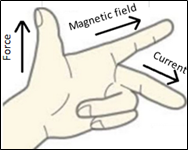## NCERT Class 10 Science : Learning Concept

### Science: Chapter 13-Magnetic Effects of Electric Current-Concepts

13.3 Force on a Current Carrying Conductor in a Magnetic Field

We have seen that a current carrying conductor creates a magnetic field. Now if a current carrying conductor is placed inside a magnetic field, the magnetic field will exert a force on the current carrying conductor.
Directions of the Force can be determined by Fleming’s Left-Hand Rule
Fleming’s Left-Hand Rule:
If we stretch the thumb, forefinger and middle finger of our left hand such that they are mutually perpendicular to each other as shown in the figure. If the first finger points in the direction of magnetic field and the second finger in the direction of current, then the thumb will point in the direction of motion or the force acting on the conductor.Figure 5: Fleming’s Left-Hand Rule